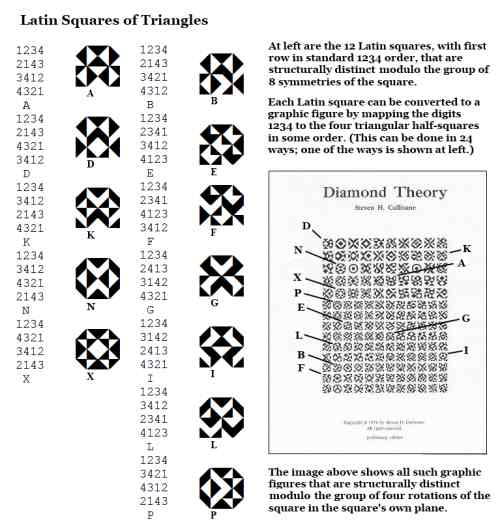Finite Geometry Notes   | Home | Site Map | Author |

The Order-4 Latin Squares

by Steven H. Cullinane, Jan. 22, 2011

The following is from the weblog of a high school mathematics teacher—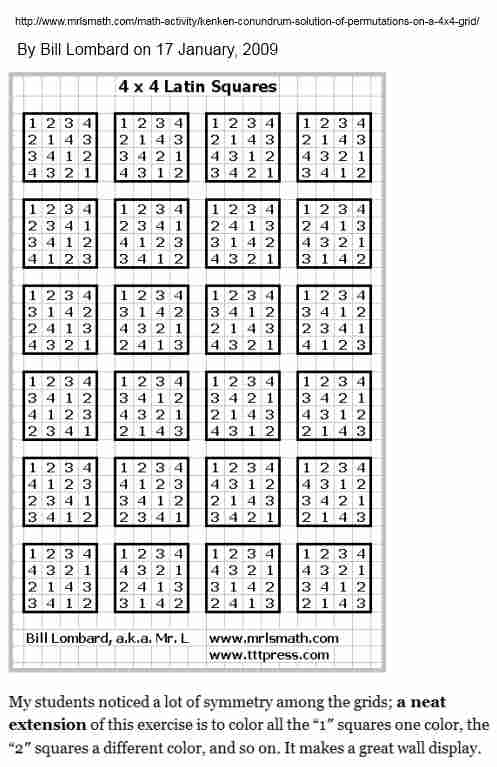This is related to the structure of the figure on the cover of the 1976 monograph Diamond Theory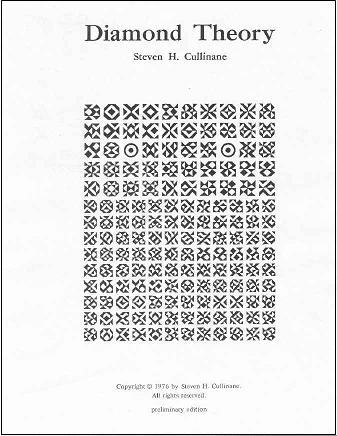Each small square pattern on the cover is a Latin square,
with elements that are geometric figures rather than letters or numerals.
All order-four Latin squares are represented.

For a deeper look at the structure of such squares, let the high-school
chart above be labeled with the letters A through X, and apply the
four-color decomposition theorem.  The result is 24 structural diagrams—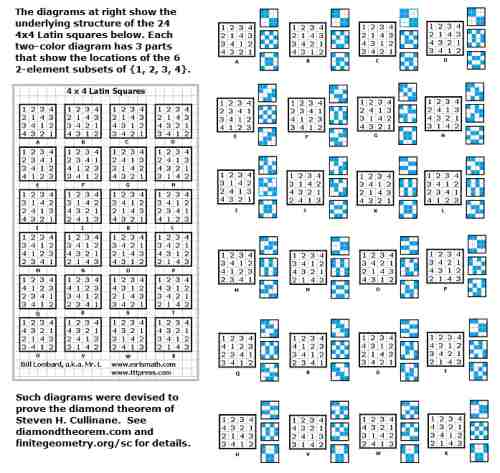Some of the squares are structurally congruent under the group of 8 symmetries of the square.

This can be seen in the following regrouping—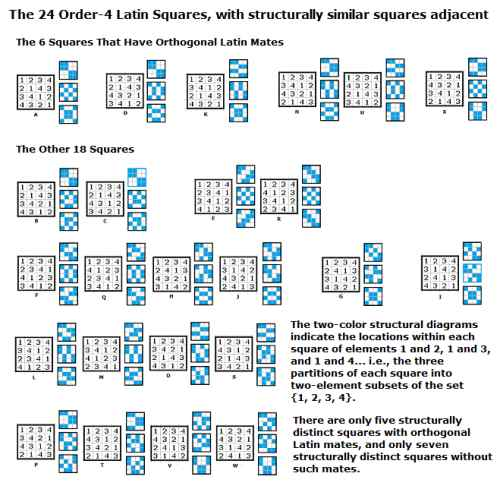(Image corrected on Jan. 25, 2011-- "seven" replaced "eight.")

Update of Feb. 5, 2011—

Click to enlarge